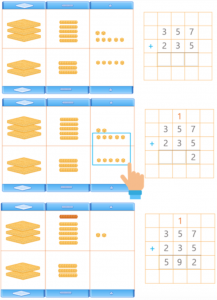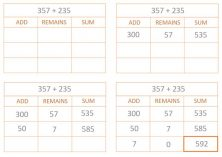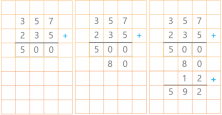Smartick is a fun way to learn math!May11

Each and every one of us has our own style of learning, and not all strategies work for everyone. In today’s entry we’re going to show you different addition algorithm, ways to solve a vertical addition with carrying, so you can choose the simplest algorithm for you.

It’s the classic addition algorithm with a manipulative visual aid to better understand carrying:The first step is to represent the addition with the blocks. The first summand is 357. We need 7 cubes (units), 5 tens (rods), and 3 hundreds (flats), and then we do the same with the second summand: 5 units, 3 tens, and 2 hundreds.

Once we’ve represented the addition with the blocks, by looking at the units we can see that, in total, we have more than 10 units. Therefore, we can select them and make a ten. Once we’ve formed the ten, we can finish adding the units. We have two units left, which we write down in the sum under the units column, and we write the ten we’ve formed in the tens column.

Then continue with the tens. We have a total of 8 tens, and when we add the one we formed, 9. Since there are fewer than 10 tens, we can’t form a hundred, so we write down the result of the sum and we move on to the tens. 3 plus 2 equals 5, so now we have the result of the addition. 357 + 235 = 592.

The blocks are a material that facilitates the understanding of positional value and carrying, and are highly recommended for learning addition.

The creator of this method is Jaime Martínez Montero, master and doctor of Philosophy and Education Sciences.First of all, write the sum in the table header. This table, as you can see, consists of 3 columns. The first column represents the quantity of the first summand that we choose to add to the second summand. The second column is what is left of the first summand, and the third column is the sum of the quantity we’ve chosen from the first summand added to the second summand.

Both the order of the columns and the quantities that we choose to add are the choice of the person who is doing the addition, depending on their calculation ability. Therefore, they can choose simpler sums and complete the process in as many steps as they need.

## Addition algorithm: Left to right method (Dutch algorithm)In this method, we start adding from the left, so we add the hundreds first: 3 hundreds plus 2 hundreds equals 5 hundreds, 500. Then we add the tens, 5 plus 3 is 8 tens, 80. Finally we add the units. Adding from the left to the to the right means that you don’t have to note down or remember any numbers you would usually carry in the more common method.

There you have it! Three ways to solve addition with carrying, now you just have to choose the one you like most!